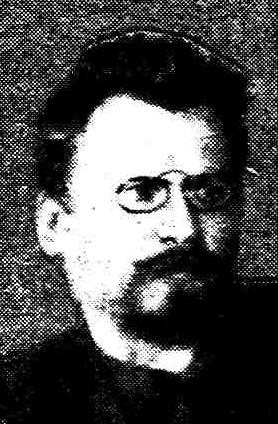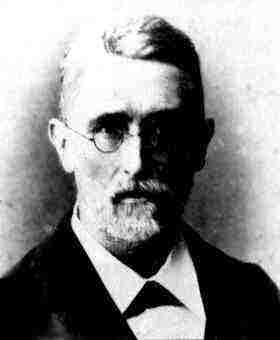# Algorithm of Voronoi in Pure Cubic Fields

Racing along the VORONOI Highway:

For the determination of the principal factorization type of a pure cubic number field L = Q(R1/3) with radicand R = mn2 we use the Algorithm of VORONOI .This is a method of finding the period of all lattice minima in the discrete embedding of the maximal order of L. The period starts with the trivial unit 1 and terminates with the fundamental unit e0 < 1 of L. The other elements of the period are algebraic integers with small norms below the Minkowski bound.

Generators of primitive ambiguous principal ideals of L are also algebraic integers with small norms. Hence there is a considerable probability to find these generators among the lattice minima of the period of the maximal order and the rare fields where this is not the case are called exotic fields. Indeed, it can be proved for extensive classes of pure cubic number fields that generators of primitive ambiguous principal ideals different from radicals must appear as lattice minima in the period of the maximal order or of a special suborder:

1. for fields of the 1st Dedekind type with radicands R congruent 0 modulo 3 in the maximal order OL = Z + Z(mn2)1/3 + Z(m2n)1/3 ,
2.3. for fields of the 2nd Dedekind type with radicands R congruent 1,-1 modulo 9 in the special suborder O = Z + Z(mn2)1/3 + Z(m2n)1/3 , but not in the maximal order OL = Z[1+m(mn2)1/3 +n(m2n)1/3] + Z(mn2)1/3 + Z(m2n)1/3.

Particularly obstinate fields:

A general statement for the maximal order of the remaining fields of Dedekind's 1st type with radicands R congruent 2,-2,4,-4 modulo 9 is not possible. For these fields a distinguished suborder exists only in the case of a quadratic part n > 1.

But in the treatment of fields with radicands R congruent 2,-2,4,-4 modulo 9 we are aided by the lucky fact that here the principal factorization type GAMMA is excluded, since the radicand R has at least one prime factor p incongruent 1,-1 modulo 9 and p <> 3. For the principal factorization types BETA and GAMMA there exists a common criterion in the form of a congruence condition for the coefficients of the fundamental unit e0. Hence we can separate the types ALPHA and BETA for R congruent 2,-2,4,-4 modulo 9 similarly as the types ALPHA and GAMMA for R congruent 0 modulo 3 and R congruent 1,-1 modulo 9. For the most recent results see my series Joint Research 2002.

Bibliography: